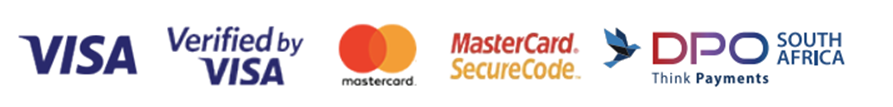﻿ Grade 7 - Singapore Maths

Singapore Maths is the generic name of the maths curriculum or syllabus that is created by the Education Ministry in Singapore for use in Singapore schools. As more and more people around the world became fascinated by the astounding success of Singapore students in mathematics, they began referring to Singapore's maths curriculum as simply Sing...

Singapore Maths is the generic name of the maths curriculum or syllabus that is created by the Education Ministry in Singapore for use in Singapore schools. As more and more people around the world became fascinated by the astounding success of Singapore students in mathematics, they began referring to Singapore's maths curriculum as simply Singapore Maths.

For each Grade there are Core and Supplementary Books. These will be indicated on the DATA SHEET for each title. Â

This system utilises Â CONCRETE - PICTORIAL - ABSTRACT . Â Manipulatives are necessary to encourage learners to visualise and solve problems.Â Please see the MANIPULATIVES & TEACHER RESOURCES catagory for what is available at the appropriate Grade level.

Â

Showing 1 - 20 of 20 items
• ##### My Pals Are Here Maths Pupil's Book 6A (3rd Edition)
R215.00

My Pals Are Here! Maths Pupil's book 6A (3rd Edition) (Textbook) used in conjunctionÂ My Pals Are Here! Maths Workbook 6A (3rd Edition) to be followed by 6B. (9789814684019)

• ##### My Pals Are Here Maths Pupil's Book 6B (3rd Edition)
R215.00

My Pals Are Here! Maths Pupil's book 6B (3rd Edition) (Textbook) used in conjunctionÂ My Pals Are Here! Maths Workbook 6B (3rd Edition) (9789813168800)

• ##### My Pals Are Here Maths Workbook 6A (3rd Edition)
R180.00

My Pals Are Here Maths 6A Workbook (3rd Edition) - used in conjunction with: My Pals Are Here Maths 6A Pupil's Book (textbook) (3rd Edition)

• ##### My Pals Are Here Maths Workbook 6B (3rd Edition)
R140.00

My Pals Are Here MathsÂ 6BÂ Workbook (3rd Edition) - used in conjunction with: My Pals Are Here MathsÂ 6BÂ Pupil's Book (textbook) (3rd Edition)

• ##### My Pals Are Here Maths 6A Homework Book (3rd Edition)
R228.00

My Pals Are Here MathsÂ 6A Homework Book - Used in conjunction with:My Pals Are Here MathsÂ 6A Pupil's Book (textbook) My Pals Are Here MathsÂ 6A Workbook

• ##### My Pals Are Here Maths 6B Homework Book (3rd Edition)
R228.00

My Pals Are Here MathsÂ 6B Homework Book - Used in conjunction with:My Pals Are Here Maths 6B Pupil's Book (textbook) My Pals Are Here Maths 6B Workbook

• ##### My Pals Are Here Maths Tests 6 (3rd Edition)
R234.00

My Pals Are Here MathsÂ TestsÂ 6 (3rd Edition) Â - used to supplement:My Pals Are Here Maths 6A Pupil's Book (3rd Edition)Â (textbook)Â My Pals Are Here Maths 6A WorkbookÂ (3rd Edition) My Pals Are Here Maths 6B Pupil's Book (3rd Edition) (textbook)Â My Pals Are Here Maths 6BÂ (3rd Edition)

R810.00

R810.00

• ##### Maths Challenge - Creative and Critical Thinking Skills 6B (Standard)
R156.00

Maths Challenge uses the power of Creative Mathematics to solve mathematical problems, which are much like puzzles that we can imagine and visualise in everyday objects such as bathroom room tiles and playing blocks. Fitting the pieces of these puzzles together is a skill that can be applied to solving problems in mathematics.

• ##### Maths Challenge - Creative and Critical Thinking Skills 6A (Advance)
R156.00

Maths Challenge uses the power of Creative Mathematics to solve mathematical problems, which are much like puzzles that we can imagine and visualise in everyday objects such as bathroom room tiles and playing blocks. Fitting the pieces of these puzzles together is a skill that can be applied to solving problems in mathematics.

• ##### Maths Challenge - Creative and Critical Thinking Skills 6B (Advance)
R156.00

Maths Challenge uses the power of Creative Mathematics to solve mathematical problems, which are much like puzzles that we can imagine and visualise in everyday objects such as bathroom room tiles and playing blocks. Fitting the pieces of these puzzles together is a skill that can be applied to solving problems in mathematics.

• ##### Mightymatics Primary 6
R170.00

Mightymatics strengthens pupils' mastery of concepts and facilitates learning.Â

• ##### Solve Non Routine Mathematics Problems Workbook 6
R180.00

Solve Non-Routine Real World Mathematics Problems is a series of six primary level activity books aimed to develop vital thinking, reasoning, communication,application and metacognitive process skills.

• ##### Conquer Thinking Skills & Heuristics for Primary 6
R168.00

In this series, the entire scope and range of primary mathematics word problems are classified into different thinking skills and heuristics.

• ##### Teaching to Mastery Mathematics: Teaching Of Proprtional Reasoning
R750.00

Teaching ofÂ Proportional ReasonongÂ - From Research to Practice.Â  Based on the Singapore Maths Approach.Â  This is a professional development title.

• ##### Teaching to Mastery Mathematics Bar Modelling: A Problem-Solving Tool
R750.00

Bar ModellingÂ  - A Problem-solving Tool.Â  From reasearch to practice - an effective Singapore Math Strategy.Â  This is a professional development title.

• ##### Teaching to Mastery Mathematics: Teaching of Fraction
R750.00

Teaching of Fractions - From Research to Practice - Based on the Singapore Maths approach.Â  This is a professional development title. Â

• ##### Teaching to Mastery Mathematics: Teaching Of Decimals
R750.00

Teaching of DecimalsÂ - From Research to Practice.Â  Based on the Singapore Maths Approach.Â  This is a professional development title.

• ##### Teaching Fraction, Ratio and Percentage Effectively
R504.00

Showing 1 - 20 of 20 items